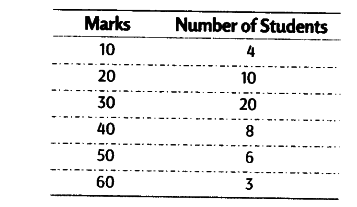# Calculate \$Q_{ 1 }\$ and \$Q_{ 3 }\$ from the following data

Calculate Q_{ 1 } and Q_{ 3 } from the following dataCalculation of Q_{ 1 } and Q_{ 3 }

Now, Q_{ 1 }= Size of (N+1/4) th item = Size of (51+1/4) th item

= Size of 13th item and it lies in cf 14, hence Q_{ 1 } = 20 marks
Q_{ 3 }= Size of 3(N+1/4) th item = Size of 3 (51+1/4) th item
= Size of 39th item and it lies in cf 42, hence Q_{ 3 } = 40 marks